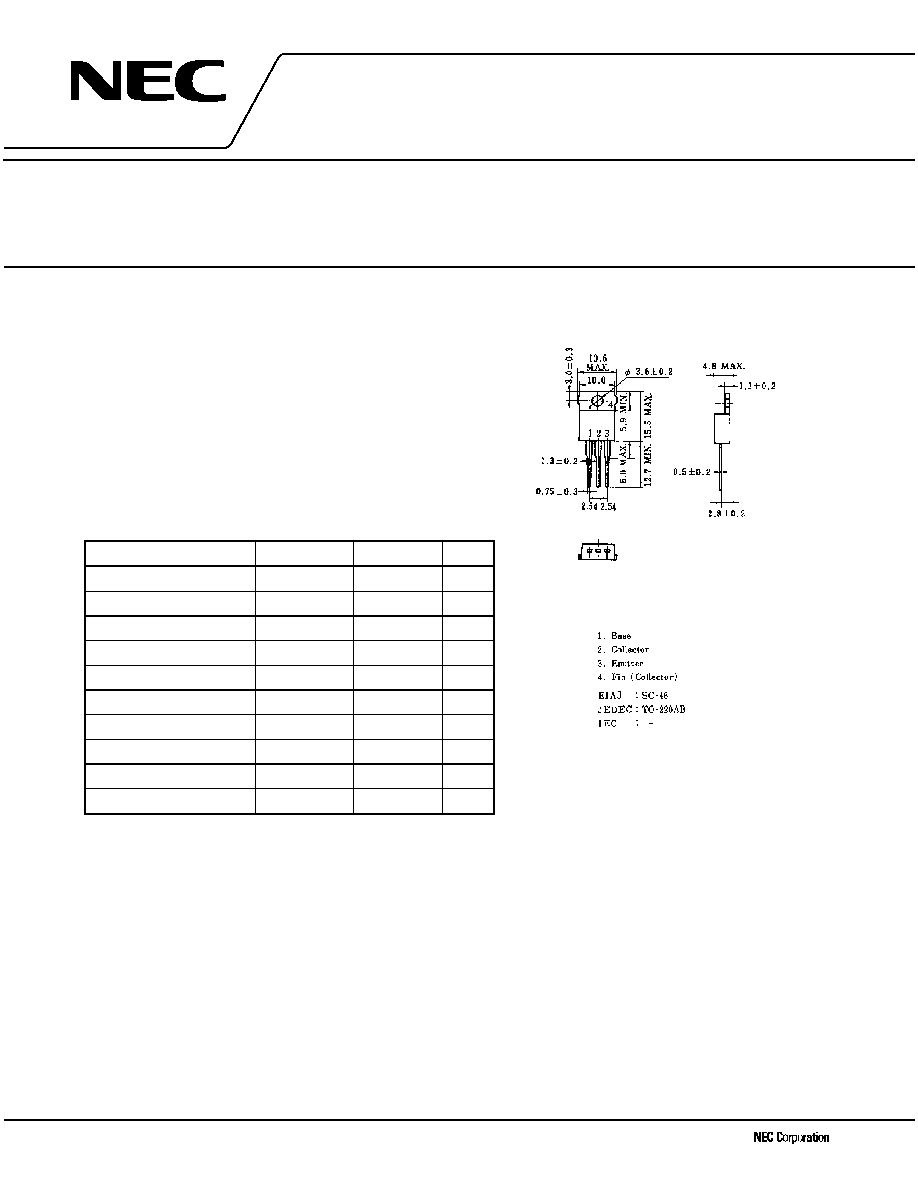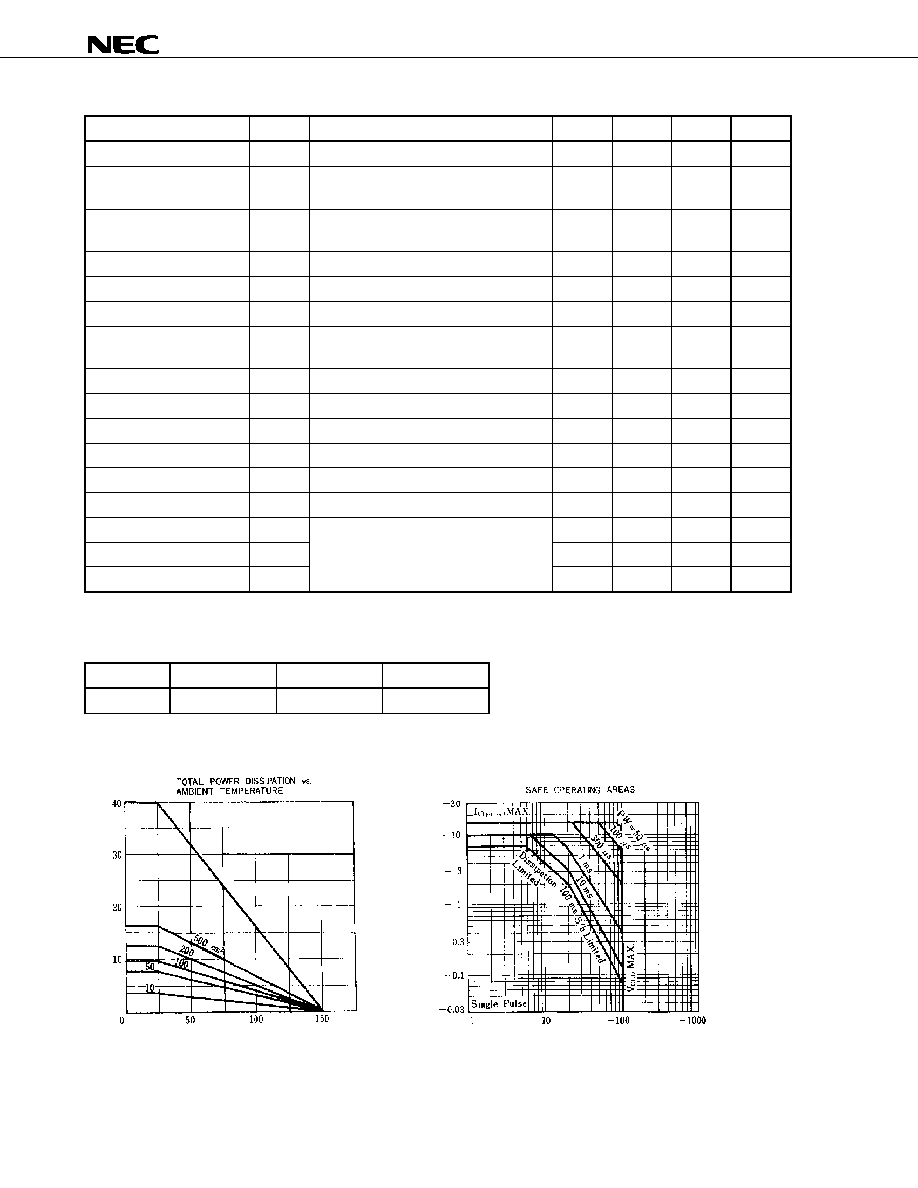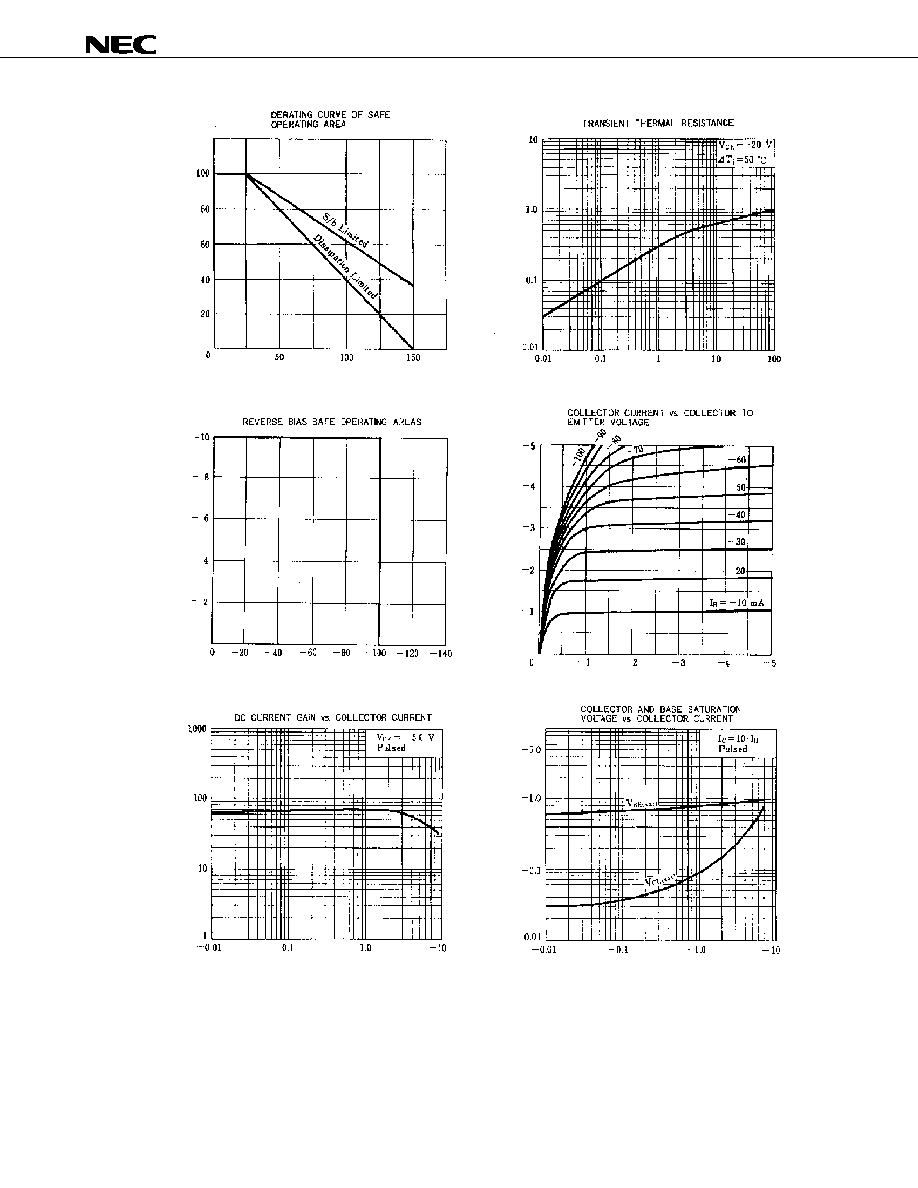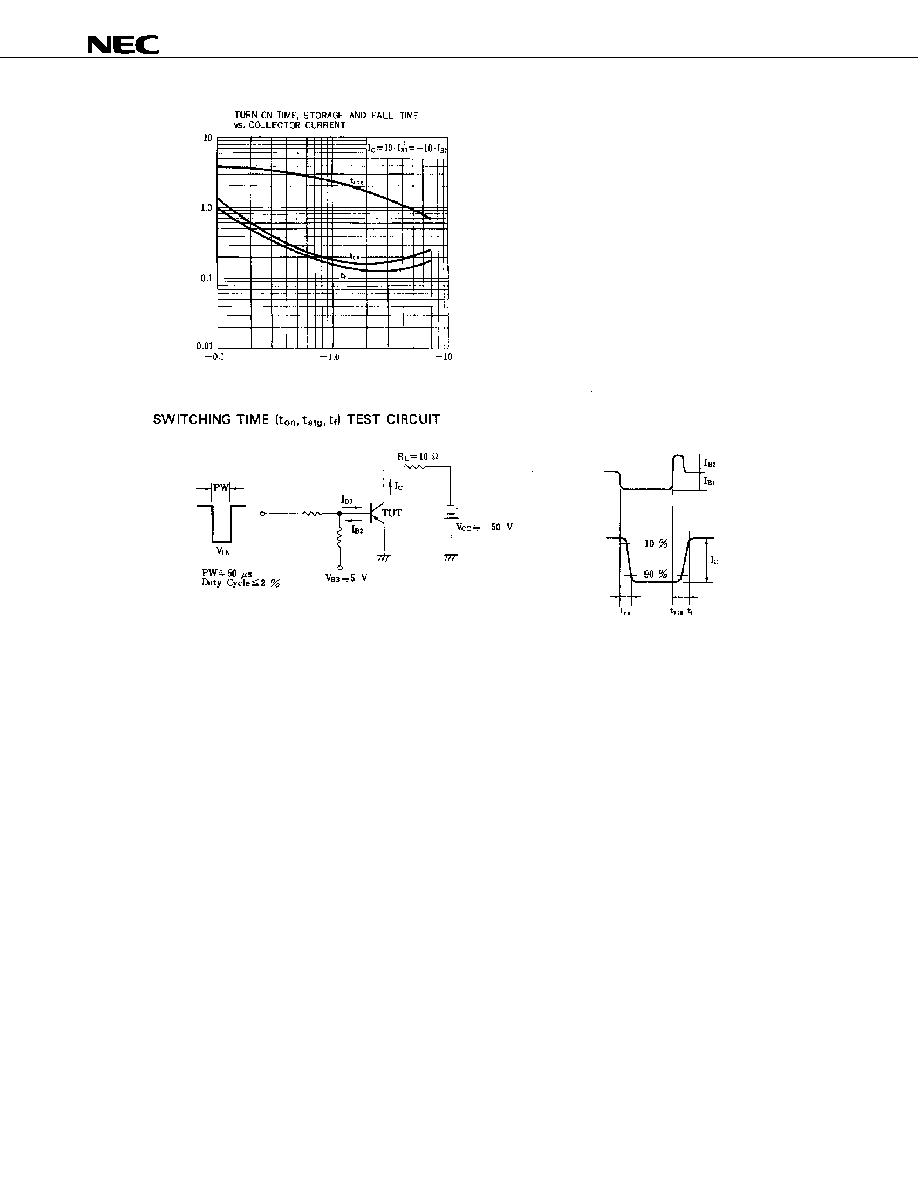Search datasheet (1.687.043 components) Search fieldPart namePart descriptionAbout site Manufacturers list ChipFindIC search engine AllXrefCross-reference database### Datasheet: 2SA1010 (NEC)

Silicon Power Transistor
Download:PDFZIP# Document Outline

• COVERDocument No. D16118EJ2V0DS00
Date Published April 2002 N CP(K)
Printed in Japan
SILICON POWER TRANSISTOR
2SA1010
PNP SILICON EPITAXIAL
TRANSISTOR
FOR HIGH-VOLTAGE HIGH-SPEED SWITCHING
DATA SHEET
®
2002
The information in this document is subject to change without notice. Before using this document, please
confirm that this is the latest version.
Not all devices/types available in every country. Please check with local NEC representative for
availability and additional information.
The 2SA1010 is a mold power transistor developed for high-
voltage high-speed switching, and is ideal for use as a driver in
devices such as switching regulators, DC/DC converters, and high-
frequency power amplifiers.
FEATURES
À Low collector saturation voltage
À Fast switching speed
À Complementary transistor: 2SC2334
ABSOLUTE MAXIMUM RATINGS (Ta = 25

C)
Parameter
Symbol
Ratings
Unit
Collector to base voltage
V
CBO
-100
V
Collector to emitter voltage
V
CEO
-100
V
Emitter to base voltage
V
EBO
-7.0
V
Collector current (DC)
I
C(DC)
-7.0
A
Collector current (pulse)
I
C(pulse)
*
-15
A
Base current (DC)
I
B(DC)
-3.5
A
Total power dissipation
P
T
(Tc = 25
░C)
40
W
Total power dissipation
P
T
(Ta = 25
░C)
1.5
W
Junction temperature
T
j
150
░C
Storage temperature
T
stg
-55 to +150
░C
* PW
300
Ás, duty cycle 10%
PACKAGE DRAWING (UNIT: mm)
Pin ConnectionData Sheet D16118EJ2V0DS
2
2SA1010
ELECTRICAL CHARACTERISTICS (Ta = 25

C)
Parameter
Symbol
Conditions
MIN.
TYP.
MAX.
Unit
Collector to emitter voltage
V
CEO(SUS)
I
C
=
-5.0 A, I
B1
=
-0.5 A, L = 1 mH
-100
V
Collector to emitter voltage
V
CEX(SUS)1
I
C
=
-5.0 A, I
B1
=
-I
B2
=
-0.5 A,
V
BE(OFF)
= 5.0 V, L = 180
ÁH, clamped
-100
V
Collector to emitter voltage
V
CEX(SUS)2
I
C
=
-10 A, I
B1
=
-1.0 A, I
B2
=
-0.5 A,
V
BE(OFF)
= 5.0 V, L = 180
ÁH, clamped
-100
V
Collector cutoff current
I
CBO
V
CB
=
-100 V, I
E
= 0
-10
ÁA
Collector cutoff current
I
CER
V
CE
=
-100 V, R
BE
= 51
, Ta = 125 ░C
-1.0
mA
Collector cutoff current
I
CEX1
V
CE
=
-100 V, V
BE(OFF)
= 1.5 V
-10
ÁA
Collector cutoff current
I
CEX2
V
CE
=
-100 V, V
BE(OFF)
= 1.5 V,
Ta = 125
░C
-1.0
mA
Emitter cutoff current
I
EBO
V
EB
=
-5.0 V, I
C
= 0
-10
ÁA
DC current gain
h
FE1
V
CE
=
-5.0 V, I
C
=
-0.5 A*
40
200
DC current gain
h
FE2
V
CE
=
-5.0 V, I
C
=
-3.0 A*
40
200
DC current gain
h
FE3
V
CE
=
-5.0 V, I
C
=
-5.0 A*
20
Collector saturation voltage
V
CE(sat)
I
C
=
-5.0 A, I
B
=
-0.5 A*
-0.6
V
Base saturation voltage
V
BE(sat)
I
C
=
-5.0 A, I
B
=
-0.5 A*
-1.5
V
Turn-on time
t
on
0.5
Ás
Storage time
t
stg
1.5
Ás
Fall time
t
f
I
C
=
-5.0 A, R
L
= 10
,
I
B1
=
-I
B2
=
-0.5 A, V
CC
-50 V
Refer to the test circuit.
0.5
Ás
* Pulse test PW
350
Ás, duty cycle 2%
h
FE
CLASSIFICATION
Marking
M
L
K
h
FE2
40 to 80
60 to 120
100 to 200
TYPICAL CHARACTERISTICS (Ta = 25

C)
T
o
t
a
l
P
o
w
e
r
Di
s
s
i
pa
t
i
on
P
T
(W)
Ambient Temperature Ta (
░C)
Collector to Emitter Voltage V
CE
(V)
Col
l
e
c
t
or Current
I
C
(
A
)
2 mm aluminum board,
no insulating board,
grease coating, natural
air cooling
With infinite heatsinkData Sheet D16118EJ2V0DS
3
2SA1010
Case Temperature T
C
(
C)
I
C
De
r
a
ti
n
g
d
T
(%
)
T
r
an
si
en
t

T
h
e
r
ma
l
R
e
si
st
a
n
c
e
t
h
(j
-c
)
(
C/W
)
Pulse Width PW (ms)
Collector to Emitter Voltage V
CE
(V)
Collector to Emitter Voltage V
CE
(V)
Co
l
l
e
c
to
r
Cu
r
r
e
n
t
I
C
(A
)
Co
l
l
e
c
to
r
Cu
r
r
e
n
t
I
C
(A
)
Collector Current I
C
(A)
Ba
s
e
S
a
t
u
r
a
ti
o
n

Vo
lta
g
e
V
BE(s
a
t
)
(V)
C
o
l
l
ec
t
o
r
S
a
t
u
r
a
t
i
o
n
V
o
l
t
ag
e
V
CE
(
s
a
t
)
(V)
Collector Current I
C
(A)
DC Cu
rr
e
n
t Ga
i
n

h
FEData Sheet D16118EJ2V0DS
4
2SA1010
Collector Current I
C
(A)
Tur
n
-
O
n Time
t
on
(
Á
s)
S
t
orageTi
m
e
t
st
g
(
Á
s)
Fa
ll T
i
me

t
f
(
Á
s)
Base current
waveform
Collector current
waveformData Sheet D16118EJ2V0DS
5
2SA1010
[MEMO]© 2019 • ICSheet• Contact form• Main page